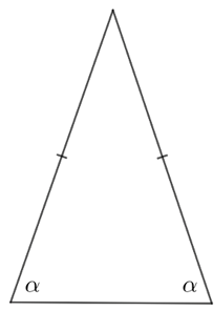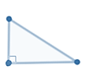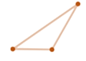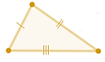PRIMARY

2 - 6

SECONDARY

7 - 10

SENIOR

11 - 12

Home

# Isosceles Triangles

Theory & Practice Questions.

## Lesson

### [Definition]

#### Isosceles Triangles

An isosceles triangle has two equal sides and two equal base angles## Example

Match the numbered statements in Column X with the letters allocated to the diagrams in Column Y by classifying according to sides or angles.

#### Column Y

##### 1

An acute triangle has 3 acute angles

A
#####2

A right triangle has 1 right angle

B
#####3

An obtuse triangle has 1 obtuse angle

C
#####4

An equilateral triangle has 3 congruent sides

D
#####5

An isosceles triangle has at least 2 congruent sides

E
#####6

A scalene triangle has no sides of equal length

F

## Solution

 1-E   All angles are less than 90° 2-A   One angles is equal to 90° 3-B   One angle is greater than 90° 4-C  All sides are of equal length 5-F   Two sides are of equal length 6-D   None of the sides are equal length

## Question

A triangle’s largest angle is 2.5 times larger than the smallest angle. If the third angle is 40°, what type of triangle is it?

## Explanation

Given the information, we can create an equation and solve.

2.5x + x +40 = 180

3.5x = 140

x = 40

Therefore, the angles are 100°, 40°, 40° and the triangle is isosceles.